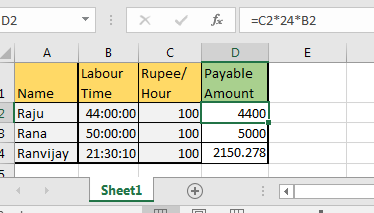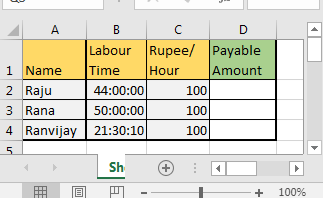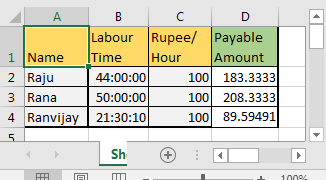# How to get the Multiplying Time Values And Numbers in ExcelHey there! You are trying to multiply time with the whole number in Excel but not getting what you want, right? This is because date and time are treated differently in Excel. Since it is easier to show than to explain it, let’s start with an example.

So let’s say, you have time and per hour pay data in excel. Now I want you to calculate the net payable amount of each entry as shown in the below image.Column A contains the names of workers.

B has the time they worked for in HH:MM:SS format.

C contains pay per hour. It is too low, I know.

In column D we want the net payable amount for that employee.

When you try to directly multiply cells in excel you will give an answer like this.For Raju it is 189.3333. Anyone can tell that it is incorrect. But why? It should be 4400, isn’t it?

Well, when a cell is formatted as date and time, 1 translates to 24 in that cell. So each value in B column is equal to “value/24”

So to get the hours as a number, we need to multiply the cell with 24 first.

When we multiply cell B2 with 24, we get 44 as the whole number. Now we can multiply it with C2 to get the correct value.

In cell D2 write this formula and copy it in cells below:

 =C2*24*B2This will work in all versions of Microsoft Excel as it is simple math.

Here I tried to explain how time and date are treated in Excel and how to do multiplication of time in excel.

So how was it? Did you learn something new? Was it helpful? Share your questions and thoughts in the comments section.

Related Articles:

Calculate Minutes Between Dates & Time In Microsoft Excel

Calculate hours between time in Excel

Excel convert decimal Seconds into time format

Popular Articles:

50 Excel Shortcuts to Increase Your Productivity

How to use the VLOOKUP Function in Excel

How to use the COUNTIF function in Excel

How to use the SUMIF Function in Excel

## Users are saying about us...

1.mathematics

2.Begin Date:02/22/19
End Date:06/30/19
Daily Rate: \$129.00
Paid Amount: \$41,796.00

*I need formula to calculate monthly from 02/22/19 to 02/28/19; 03/01/19 to 03/31/19 and so on.
**The information above are in columns

•It is simple Airen Greene. Just subtract the start date from the end date and multiply it by the daily rate. For example, if start date is in a2 and end date is in b2 and daily rate 129 then below formula will give the total payable ammount.
=(B2-A2)*129

3.In one Cell Time addition suppose =01:00+2:00 it will calculate 03:00 please tell me how to do that

•1 hour in excel is equal to 1/24. So if you want to add 3 hours to a clock time then do it like this. time+3x(1/24). this will add 3 hours to the given time.
but if you have time in time format (hh:mm:ss) then the simple addition would work (time + time).

4.I get paid by the minute. What would be the formula to say multiply 3:10:15 x \$.90 ?

5.Clearly explained - worked for me. Thank you

•Thanks Elise.

Terms and Conditions of use

The applications/code on this site are distributed as is and without warranties or liability. In no event shall the owner of the copyrights, or the authors of the applications/code be liable for any loss of profit, any problems or any damage resulting from the use or evaluation of the applications/code.